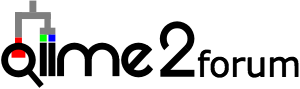# Import Data- NovaSeq sequencing data. Error message in not recognizing forward.fastq.gz file

Hey team! I have run into a problem when importing my data. My sequencing data was sequenced at a sequencing facility on a Illumina NovaSeq. I renamed my fastq files from their given name of something like 'Pool1_X28947392_L1_1.fq' to 'forward.fastq.gz' and same for the reverse read. I also have my metadata.tsv file with the correct barcodes. Then when I ran the first command recommended in the import:

qiime tools import
--type MultiplexedPairedEndBarcodeInSequence
--input-path Pool_1
--output-path original_seqs.qza

'Missing one or more files for MultiplexedPairedEndBarcodesInSequenceDirFmt: 'forward.fastq.gz''

Is this error likely due to the formatting of the fastq file? It was originally a .fq file so I am not sure if this changes it. I was trying to figure out why others are getting this error as well and it keeps coming back to making sure the format is correct. I have checked the format:

The file looks something like this:

@A01415:38:H2FM3DRXY:1:2101:2483:1031 1:N:0:CTATGCCT+AAGAGGCA
ANTGATACGGCGACCACCGAGATCTACACGCTTGATATCGTCTTTATGGTAATTGTGTGTCAGCAGCCGCGGTAATACGGGGGGGACAAGTGTTATTCGGAATGACTGGGCGTAAAGAGTCTGTAGGCGGTTTTTTAAGTTGAATGCTAAAACTTGGATCTCAATTCCAAGAAGATGTTCAAAACTGATTAACTAGAGATTGAGAGGGGACAGTAGAATTTCTAATGGAGAGATAAAATTCATAGATATT
+
F#FFFFFFFFFFFFFF:FFFFFFFFFFFFFF:FFFF:FFFFFFFFF::FF,FF,FFFFFFFFFFFFFFFFFFFF:FFFFFFFFFFFFFFFFFFFFFFFFFF:FFF:,FFFFFFFFFFFFFFFFFFFFF,FFFFFFFFFFFFFFFFFFFFFFFFFFFFFFFFFF:FF:FFFFFFFFFFFFF:FFF,FFFFF:FF:FF,::FFFFFFF:FF:FFFFFFFFFFFFF:F:,FFFFFFFFFFFFFFFFFFFFFFF
@A01415:38:H2FM3DRXY:1:2101:9263:1031 1:N:0:CTATGCCT+AAGAGGCA
ANTGATACGGCGACCACCGAGATCTACACGCTGACTCAACCAGTTATGGTAATTGTGTGCCAGCAGCCGCGGTAAAACCAGCACCTCAAGTGGTCAGGATGATTATTGGGCCTAAAGCATCCGTAGCCGGCTCTGTAAGTTTTCGGTTAAATCTGTACGCTCAACGTACAGGCTGCCGGGAATACTGCAAAGCTAGGGAGTGGGAGAAGTAGACGGTACTCGGTAGGAAGTGGTAAAATGCTTTGATCTA
+
:#FFFFFFFFFFFFFFFFFFFFFFFFFFFFFFFFFFFFFFFFFFFF:FFFFFFFFFFFFFFFFFFFFFFFFFFFFFFFFFFFFFFFFFFFFFFFFFFFF,FFF:::FFFF:FFFFFFF:FFFFFF,FFFFFFFFFFFFFFFFFFF:FFF,FFFF:FFFFFFF:,FFFFF,FFFFFF:FFFF:FFFFF:FFFF:FF,FFFF,FFFFF,FFFFFFFFFFF:FFFF,FFF,FFFFFF,FF,FFF:FFF:FFFF

There are 45ish samples in each Pool. So Pool 1 should have 45 different barcoded samples in it. The primer and the barcode is still in the sequence

For example the sequence in the first one is:

sequence wanted: TACGGGGGGGACAAGTGTTATTCGGAATGACTGGGCGTAAAGAGTCTGTAGGCGGTTTTTTAAGTTGAATGCTAAAACTTGGATCTCAATTCCAAGAAGATGTTCAAAACTGATTAACTAGAGATTGAGAGGGGACAGTAGAATTTCTAATGGAGAGATAAAATTCATAGATATT

My primers are:

Golay barcode: XXXXXXXXXXXX
Forward primer (515F): GTGYCAGCMGCCGCGGTAA

Reverse complement of 3′ Illumina adapter: CAAGCAGAAGACGGCATACGAGAT